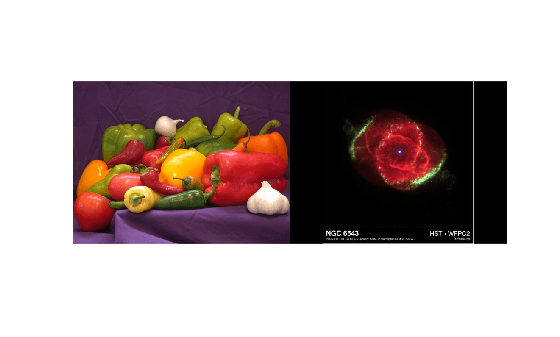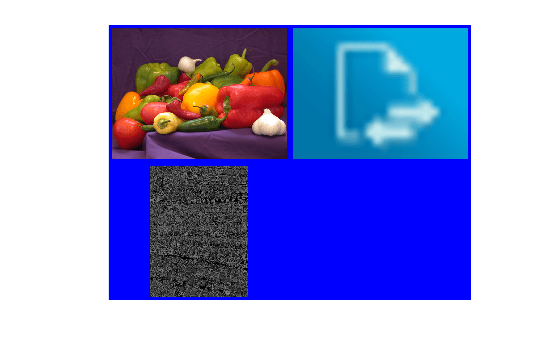# imtile

Combine multiple image frames into one rectangular tiled image

## Syntax

``out = imtile(filenames)``
``out = imtile(I)``
``out = imtile(images)``
``out = imtile(imds)``
``out = imtile(X,map)``
``out = imtile(___,Name,Value)``

## Description

``` `out = imtile(filenames)` returns a tiled image containing the images specified in `filenames`. `filenames` is an n-by-1 or 1-by-n string array, character vector, or cell array of character vectors. If the files are not in the current folder or in a folder on the MATLAB® path, specify the full path name. See the `imread` command for more information. By default, `imtile` arranges the images so that they roughly form a square, but you can change that using optional parameters. The images can have different sizes and types. If you specify an indexed image, then `imtile` converts it to RGB using the colormap present in the file.If there is a data type mismatch between images, then `imtile` rescales all images to be `double` using the `im2double` function.```
````out = imtile(I)` returns a tiled image containing all the frames of the multiframe image array `I`. A multiframe image array can be a sequence of binary, grayscale, or truecolor images. ```
``` `out = imtile(images)` returns a tiled image containing the images specified in the cell array `images`. `imtile` displays empty cell array elements as a blank tile.```
``` `out = imtile(imds)` returns a tiled image containing the images specified in the ImageDatastore object `imds`. For information about image datastores, see `ImageDatastore`. ```
````out = imtile(X,map)` treats all grayscale images in `X` as indexed images and applies the specified colormap `map` to all frames. `X` can be an array of grayscale images (m-by-n-by-1-by-k), a string array of file names, or a cell array of character vectors. If `X` represents file names, `map` overrides any internal colormap present in the image files.```
````out = imtile(___,Name,Value)` returns a customized tiled image, depending on the values of the optional parameter name-value pairs. You can abbreviate parameter names, and case does not matter.```

## Examples

collapse all

Read multiple images from files into the workspace and create a tiled image containing the images. Display the tiled image.

```out = imtile({'peppers.png', 'ngc6543a.jpg'}); imshow(out);```Using a data set containing multiple images, tile the images in a grid.

```load mri out = imtile(D, map); imshow(out);```Create a tiled image containing only the first eight images in the data set. Use the `'GridSize'` parameter to arrange the images in a 2-by-4 grid.

```out = imtile(D, map, 'Frames', 1:8, 'GridSize', [2 4]); figure; imshow(out);```Read an RGB image into the workspace.

`imRGB = imread('peppers.png');`

Create a tiled image containing each of the three planes of the RGB image. Display the tiled image.

```out = imtile(imRGB); imshow(out)```From an image datastore, create and customize a tiled image.

Create an image datastore containing all the files with the file extension `'tif'` or `'png'` in the specified folder.

```fileFolder = fullfile(matlabroot,'toolbox','matlab','imagesci'); imds = imageDatastore(fileFolder,'FileExtensions',{'.tif','.png'});```

Create a tiled image containing the images in the datastore.

```out1 = imtile(imds); imshow(out1);```Use the `'BorderSize'` and '`BackgroundColor'` parameters to add a blue border to the tiled image.

```out2 = imtile(imds, 'BorderSize', 10, 'BackgroundColor', 'b'); figure; imshow(out2);```## Input Arguments

collapse all

Names of files containing images, specified as an n-by-1 or 1-by-n string array, character vector, or cell array of character vectors.

Multiframe image, specified as a sequence of binary, grayscale, or truecolor images. `I` can be an m-by-n-by-k or an m-by-n-by-1-by-k array, or a sequence of binary, grayscale, or truecolor images. A binary or grayscale image sequence must be an m-by-n-by-k or an m-by-n-by-1-by-k array. A truecolor image sequence must be an m-by-n-by-3-by-k array.

List of images, specified as an n-by-1 or 1-by-n cell array of numeric matrices. The cell array can contain numeric matrices of size m-by-n or m-by-n-by-3.

Image data store, specified as an ImageDatastore object.

Indexed image and associated colormap, specified as a 2-D numeric array (image) and an n-by-3 numeric array of class `double` (colormap). n is the number of colors in the colormap.

### Name-Value Pair Arguments

Specify optional comma-separated pairs of `Name,Value` arguments. `Name` is the argument name and `Value` is the corresponding value. `Name` must appear inside quotes. You can specify several name and value pair arguments in any order as `Name1,Value1,...,NameN,ValueN`.

Example: ```tiled_image = imtile({'peppers.png', 'ngc6543a.jpg'},'BackgroundColor','g'); ```

Color of the background, specified as the comma-separated pair consisting of `'BackgroundColor'` and MATLAB `ColorSpec (Color Specification)`. The `imtile` function fills all blank spaces with this color, including the space specified by `BorderSize`. If you specify a background color, the `imtile` function renders the output as an RGB image.

Example: `'green'`

Example: `'g'`

Example: `[0 1 0]`

Padding around each thumbnail image, specified as the comma-separated pair consisting of `'BorderSize'` and a numeric scalar or 1-by-2 vector of the form `[brows bcols]`. `imtile` pads the borders with the background color.

Frames to include, specified as the comma-separated pair consisting of `'Frames'` and a numeric array or a logical mask. The `imtile` function interprets the values as indices into the image array or cell array. The following examples create a tiled image containing the first three image frames.

Example: `out = imtile(I,'Frames',1:3);`

Example: ```out = imtile(I,'Frames',[true true true]);```

Number of rows and columns of thumbnails in tiled image, specified as the comma-separated pair consisting of `'GridSize'` and a two-element vector of the form `[nrows ncols]`. `nrows` specifies the number of rows in the grid and `ncols` specifies the number of columns in the grid. Use `NaN`s or `Inf`s to have `imtile` calculate the size in a particular dimension in a way that includes all the images.

• If `'GridSize'` is `[2 NaN]`, then `imtile` creates a tiled image with two rows and the number of columns necessary to include all the images.

• If both the elements are `NaN` or `Inf`, then `imtile` calculates the grid size to form a square. `imtile` returns the images horizontally across columns.

• If there is a mismatch between `GridSize` and number of images (frames), `imtile` creates the tiled image based on `GridSize`.

Size of thumbnails, specified as the comma-separated pair consisting of `'ThumbnailSize'` and a two-element vector of the form `[trows tcols]`, in pixels. The `imtile` function preserves the aspect ratio of the original image by zero-padding the boundary.

• If you specify a `NaN` or `Inf`, then the `imtile` function calculates the corresponding value automatically to preserve the aspect ratio of the first image.

• If you specify an empty array (`[]`), then the `imtile` function uses the full size of the first image as the thumbnail size.

## Output Arguments

collapse all

Tiled output image, returned as a numeric array.